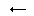# Sliders Puzzle, Proof of solvability

Here I shall prove a claim made elsewhere regarding the slider puzzles.

On a torus, every 4x4 configuration is solvable.

First, let's establish the following

### Lemma

Let n = 4. For any two adjacent counters there exists a sequence of moves that leaves all counters but the given two untouched. The two given counters, as the result of this sequence of moves, swap their locations.

### Proof of the PropositionLet's show how to swap two counters a and b without disturbing all the rest. One (horizontal) move is needed to slide a into position d one column to the right from b. With the second (vertical) move we can slide a into position c adjacent to d. Now we swap counters at c and d. Next we execute reverses of the original moves; first vertical and then horizontal.

Since one can just swap any two adjacent counters one can also swap any two counters which are not necessarily adjacent. Thus the Lemma renders the Proposition obvious. First swap the counter #1 with whichever occupies its rightful location in the upper left corner. Locate the counter #2 and swap it into its position, next to the #1, and so on.### Proof of the Lemma

Since the proof is constructive, i.e. I am going to describe the sequence of moves that proves the Lemma, the main difficulty is in inventing concise notations to describe the sequence. Let's agree in advance that the sequence consisting of two moves A and B executed consecutively will be denoted AB. Now for the notations:

 S-i _slide the i-th row left S+i _slide the i-th row right Sj- _slide the j-th column up Sj+ _slide the j-th column down V(-i, j-) S-iSj+S+iSj-rotate three counters. (i,j) is in the right corner H(-i, j+) Sj+S+iSj-S-irotate three counters. (i,j) is in the right corner V(+i, j-) S+iSj+S-iSj-rotate three counters. (i,j) is in the right corner H(+i, j+) Sj+S-iSj-S+irotate three counters. (i,j) is in the right corner G(-i, j) H(-i, j+)V(-i, j-)rotate three counters. (i,j) is in the middle G(+i, j) H(+i, j+)V(+i, j-)rotate three counters. (i,j) is in the middle F(i, j, j+1) G(+i, j+1)G(i, j)S-iswap two counters. (i,j) is on the left

F(i, j, j+1), the last transformation in the table, swaps two horizontally adjacent counters. Rotating the tray 90o and applying the same transformation we'll get a sequence of moves that flips two vertically adjacent counters. This proves the Lemma.

Note that the sequences in the table produce the desired effect (diagrams) for any n > 2, not necessarily 4. However, the last line, the transformation F is specific to the 4x4 puzzle. This is due to the fact that the last move in the sequence, S-i, for n > 4, would rotate a row thus shifting (n - 4) counters out of their original locations.

Note also that the proof, although constructive, is far from optimal. With little ingenuity it's always possible to find a shorter solution.

Lastly, for n = 3, F is an identical transformation. This shows that the group of sliding transformations is not free.

### Remarks

The theory above applies only to the Sliders on torus. Don Greenwell offers different sequences of moves that cover the cases of n > 4 and even the Klein bottle and the projective plane cases.